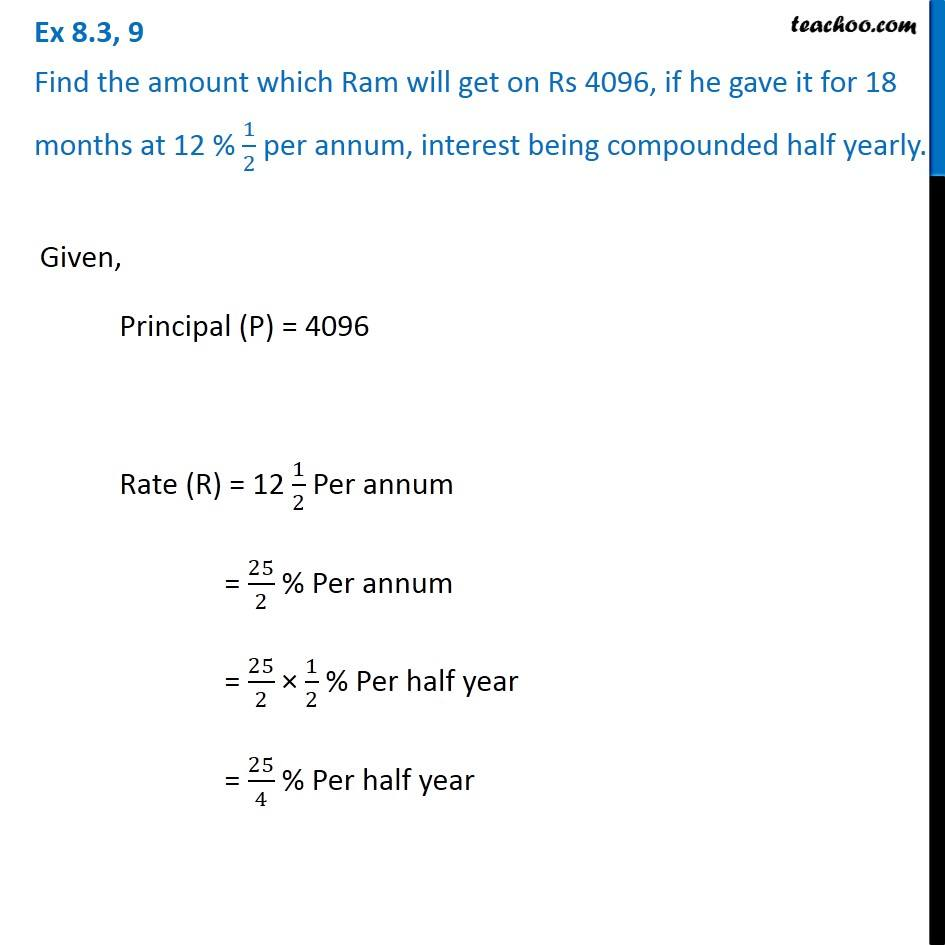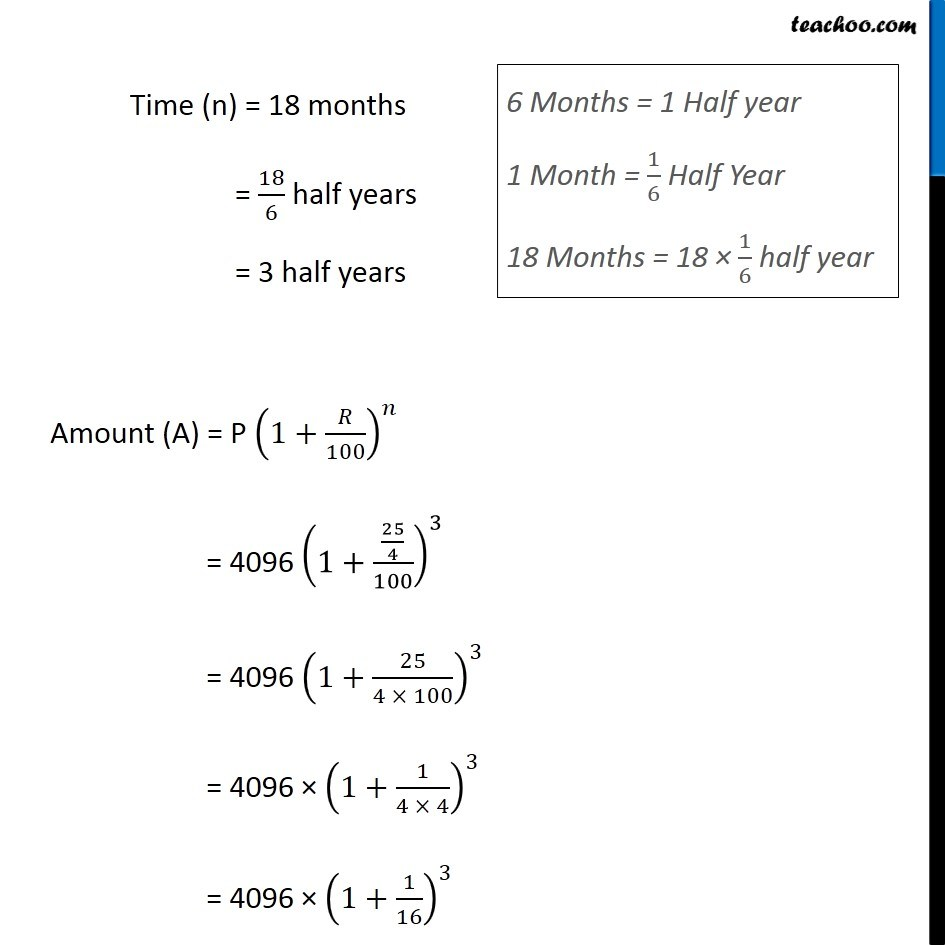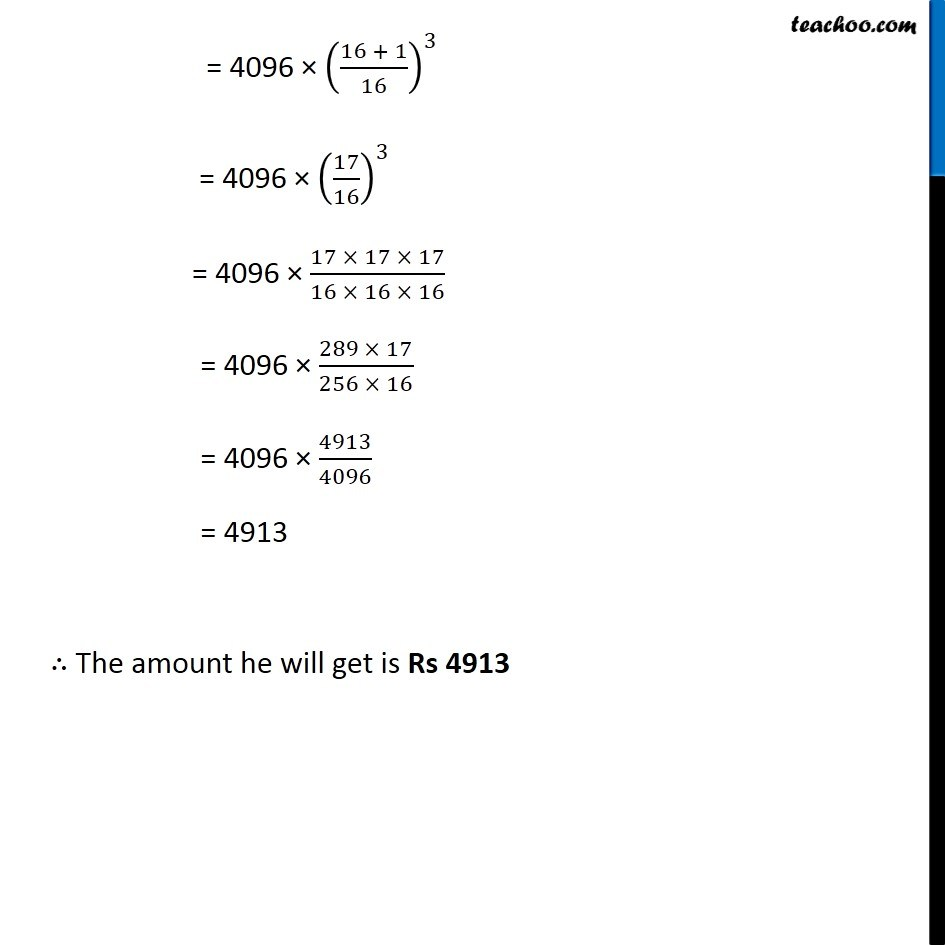Subscribe to our Youtube Channel - https://you.tube/teachoo

1. Chapter 8 Class 8 Comparing Quantities
2. Concept wise
3. Compound Interest compounded half yearly

Transcript

Ex 8.3, 9 Find the amount which Ram will get on Rs 4096, if he gave it for 18 months at 12 % 1/2 per annum, interest being compounded half yearly. Given, Principal (P) = 4096 Rate (R) = 12 1/2 Per annum = 25/2 % Per annum = 25/2 × 1/2 % Per half year = 25/4 % Per half year Time (n) = 18 months = 18/6 half years = 3 half years Amount (A) = P (1+𝑅/100)^𝑛 = 4096 (1+(25/4)/100)^3 = 4096 (1+25/(4 × 100))^3 = 4096 × (1+1/(4 × 4))^3 = 4096 × (1+1/16)^3 6 Months = 1 Half year 1 Month = 1/6 Half Year 18 Months = 18 × 1/6 half year = 4096 × ((16 + 1)/16)^3 = 4096 × (17/16)^3 = 4096 × (17 × 17 × 17)/(16 × 16 × 16) = 4096 × (289 × 17)/(256 × 16) = 4096 × 4913/4096 = 4913 ∴ The amount he will get is Rs 4913

Compound Interest compounded half yearly